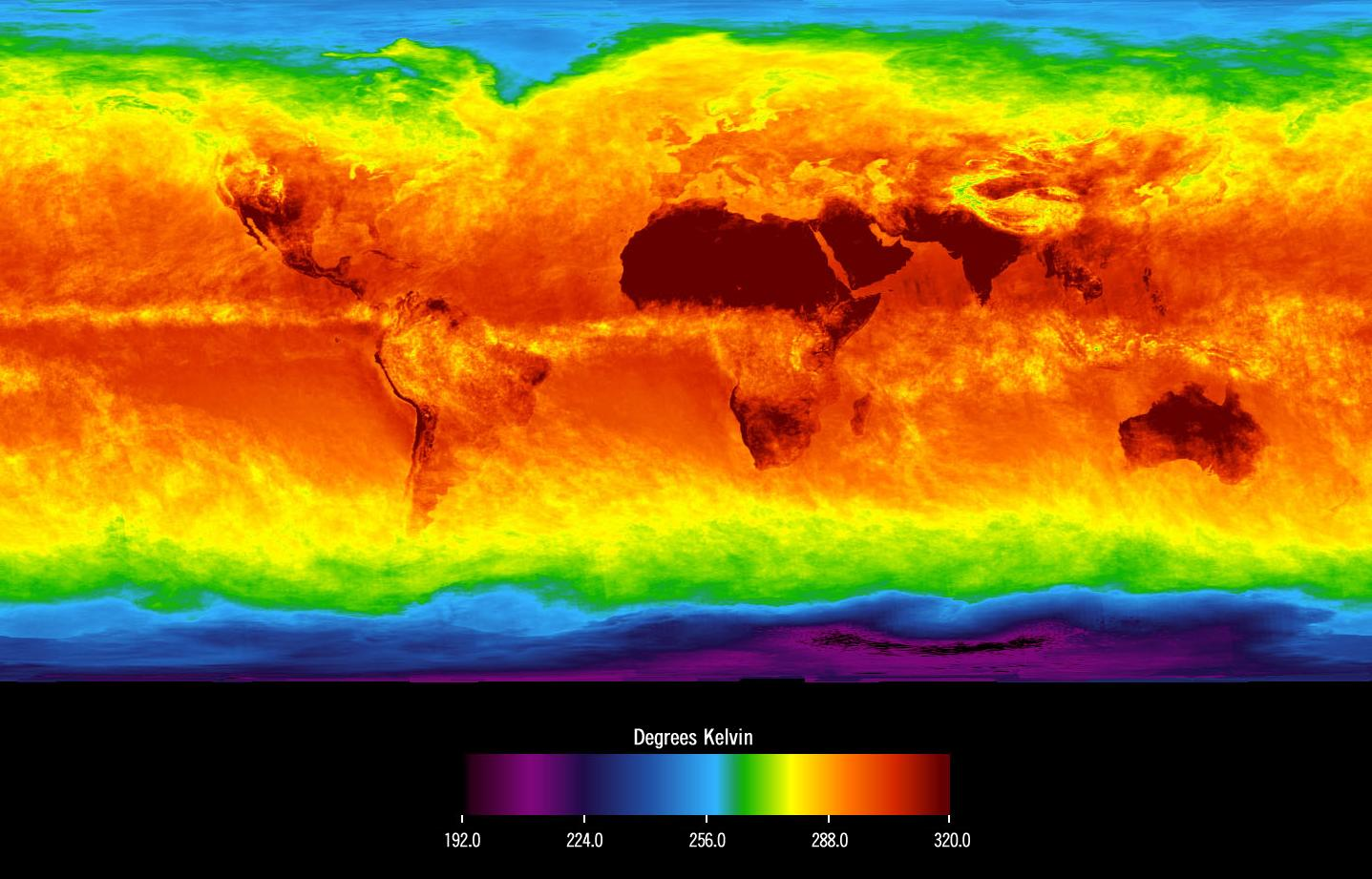### Alternative Carbon Dioxide CalculationCredit: AIRS, the Atmospheric InfraRed Sounder

After finding another paper on this subject I thought I would write a quick follow up to the previous post on the simple greenhouse model. This paper used a climate model to calculate the surface temperature of a planet with varying incident illumination and carbon dioxide partial pressure. The resulting graph is probably a simpler way for people to determine the level of the carbon dioxide necessary to meet their desired aim.

To keep this brief, the paper is called Carbonate-silicate cycle predictions of Earth-like planetary climates and testing the habitable zone concept and it investigates the connection between weather, carbon dioxide levels and surface temperature. However, most importantly, within the paper they provide an empirical equation for the mean global surface temperature for a given carbon dioxide level and incident solar flux. This equation can be used to produce a simple graph which links those three values. This chart can then be used to define the level of carbon dioxide required to achieve a certain temperature for a planet at a certain distance as desired.

Unfortunately, the equation produced in that paper is not an elegant physical model but is just a brute force numerical fit to the data.  This means it is a little unwieldy and you may wish to avert your eyes...

\begin{align}T_s(S, pCO_2) =\ &4.809 - 222.0X - 68.44X^2 - 6.737X^3 - 0.206X^4\\&+ 1414XY + 446.4X^2Y + 44.41X^3Y + 1.364X^4Y\\&- 2964XY^2 - 978.4X^2Y^2 - 98.86X^3Y^2 - 3.059X^4Y^2\\&+ 2655XY^3 + 907.5X^2Y^3 + 92.87X^3Y^3 + 2.892X^4Y^3\\&- 868.4XY^4 - 304.6X^2Y^4 - 31.48X^3Y^4 - 0.985X^4Y^4\\&+ 1045Y - 1496Y^2 + 1064Y^3 - 281.1Y^4\end{align}

$$X = \ln(pCO_2)$$, $$pCO_2$$ is the partial pressure of carbon dioxide in bar and $$Y$$ is the incident stellar flux relative to that received by Earth (i.e. divided by the solar constant). Also, it is important to note that this relationship is only valid for planets orbiting within the habitable zone of sun-like stars

Now, this equation is actually more than a little unwieldy. If you know the carbon dioxide level and the incident flux then you can calculate the surface temperature. You probably don't want to do that by hand though! However, if you want to calculate what level of carbon dioxide is needed to achieve a particular temperature, well, that's rather more difficult.

Conveniently, I have produced a graph that shows the constant temperature curves for a range of surface temperatures. Using this you can define the incident stellar flux (or the distance around the sun-like star) and the temperature you want your planet to be then just look up the approximate carbon dioxide partial pressure.

Using this chart is somewhat easier than the method described in the previous post and it is probably sufficiently accurate for most world building purposes as it will allow a ball park estimate of the carbon dioxide level to achieve a desired surface temperature for a given stellar flux. This is much quicker and perfectly adequate for world building. It is however instructive to delve into the details discussed in the previous post if you are not afraid of the equations.

After those two equation heavy posts I will try to write some softer articles next to provide some relief. I am still unsure where the best balance is for people reading this blog, so please do let me know whether the equations are helpful or just too much.

If you found this post informative then please take the opportunity to subscribe via the link at the top of the page to receive an email when new articles are posted. Also, be sure to comment below with your thoughts on the article and share it with anyone else who is interested in world building.